NEET  >  31 Year NEET Previous Year Questions: System of Particles & Rotational Motion - 2

# 31 Year NEET Previous Year Questions: System of Particles & Rotational Motion - 2

Test Description

## 30 Questions MCQ Test Physics Class 11 | 31 Year NEET Previous Year Questions: System of Particles & Rotational Motion - 2

31 Year NEET Previous Year Questions: System of Particles & Rotational Motion - 2 for NEET 2023 is part of Physics Class 11 preparation. The 31 Year NEET Previous Year Questions: System of Particles & Rotational Motion - 2 questions and answers have been prepared according to the NEET exam syllabus.The 31 Year NEET Previous Year Questions: System of Particles & Rotational Motion - 2 MCQs are made for NEET 2023 Exam. Find important definitions, questions, notes, meanings, examples, exercises, MCQs and online tests for 31 Year NEET Previous Year Questions: System of Particles & Rotational Motion - 2 below.
Solutions of 31 Year NEET Previous Year Questions: System of Particles & Rotational Motion - 2 questions in English are available as part of our Physics Class 11 for NEET & 31 Year NEET Previous Year Questions: System of Particles & Rotational Motion - 2 solutions in Hindi for Physics Class 11 course. Download more important topics, notes, lectures and mock test series for NEET Exam by signing up for free. Attempt 31 Year NEET Previous Year Questions: System of Particles & Rotational Motion - 2 | 30 questions in 60 minutes | Mock test for NEET preparation | Free important questions MCQ to study Physics Class 11 for NEET Exam | Download free PDF with solutions
 1 Crore+ students have signed up on EduRev. Have you?
31 Year NEET Previous Year Questions: System of Particles & Rotational Motion - 2 - Question 1

### A solid sphere of radius R is placed on a smooth horizontal surface. A horizontal force F is applied at height h from the lowest point. For the maximum acceleration of the centre of mass, 

Detailed Solution for 31 Year NEET Previous Year Questions: System of Particles & Rotational Motion - 2 - Question 1

As friction is absent at the point of contact,

Accelerati on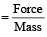It is independent of h

31 Year NEET Previous Year Questions: System of Particles & Rotational Motion - 2 - Question 2

### A boy suddenly comes and sits on a circular rotating table. What will remain conserved? 

Detailed Solution for 31 Year NEET Previous Year Questions: System of Particles & Rotational Motion - 2 - Question 2

As net torque applied is zero. Hence,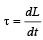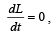, L = constant.

L (angular momentum) remains conserved.

31 Year NEET Previous Year Questions: System of Particles & Rotational Motion - 2 - Question 3

### A ball rolls without slipping. The radius of gyration of the ball about an axis passing through its centre of mass is K. If radius of the ball be R, then the fraction of total energy associated with its rotational energy will be [2003

Detailed Solution for 31 Year NEET Previous Year Questions: System of Particles & Rotational Motion - 2 - Question 3

Rotational energy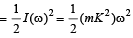Linear kinetic energy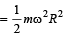∴ Required fraction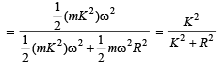31 Year NEET Previous Year Questions: System of Particles & Rotational Motion - 2 - Question 4

A solid cylinder of mass M and radius R rolls without slipping down an inclined plane of length L and height h. What is the speed of its centre of mass when the cylinder reaches its bottom ? 

Detailed Solution for 31 Year NEET Previous Year Questions: System of Particles & Rotational Motion - 2 - Question 4

Applying principle of conservation of total mechanical energy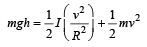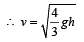31 Year NEET Previous Year Questions: System of Particles & Rotational Motion - 2 - Question 5

A thin circular ring of mass M and radius r is rotating about its axis with a constant angular velocity ω. Four objects each of mass m, are kept gently to the opposite ends of two perpendicular diameters of the ring. The angular velocity of the ring will be 

Detailed Solution for 31 Year NEET Previous Year Questions: System of Particles & Rotational Motion - 2 - Question 5

Applying conservation law of angular momentum, I1ω1 = I2ω2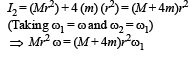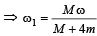31 Year NEET Previous Year Questions: System of Particles & Rotational Motion - 2 - Question 6

A wheel having moment of inertia 2 kg-m2 about its vertical axis, rotates at the rate of 60 rpm about this axis. The torque which can stop the wheel’s rotation in one minute would be 

Detailed Solution for 31 Year NEET Previous Year Questions: System of Particles & Rotational Motion - 2 - Question 6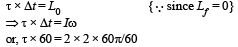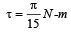31 Year NEET Previous Year Questions: System of Particles & Rotational Motion - 2 - Question 7

Consider a system of two particles having masses m1 and m2 . If the particle of mass m1 is pushed towards the centre of mass of particles through a distance d, by what distance would the particle of mass m2 move so as to keep the centre of mass of particles at the original position? 

Detailed Solution for 31 Year NEET Previous Year Questions: System of Particles & Rotational Motion - 2 - Question 7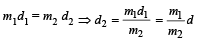31 Year NEET Previous Year Questions: System of Particles & Rotational Motion - 2 - Question 8

The ratio of the radii of gyration of a circular disc about a tangential axis in the plane of the disc and of a circular ring of the same radius about a tangential axis in the plane of the ring is 

Detailed Solution for 31 Year NEET Previous Year Questions: System of Particles & Rotational Motion - 2 - Question 8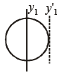Circular disc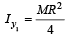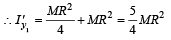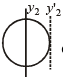Circular ring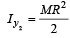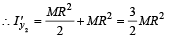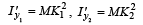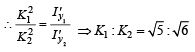31 Year NEET Previous Year Questions: System of Particles & Rotational Motion - 2 - Question 9

A r ound disc of moment of in ertia I2 about its axis perpendicular to its plane and passing through its centre is placed over another   disc of moment of inertia I1 rotating with an angular velocity ω about the same axis. The final angular velocity of the combin ation of discs is 

Detailed Solution for 31 Year NEET Previous Year Questions: System of Particles & Rotational Motion - 2 - Question 9

Angular momentum will be conserved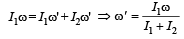31 Year NEET Previous Year Questions: System of Particles & Rotational Motion - 2 - Question 10

Three particles, each of mass m gram, are situated at the vertices of an equilateral triangle ABC of side l cm (as shown in the figure). The moment of inertia of the system about a line AX perpendicular to AB and in the plane of ABC, in gram-cm2 units will be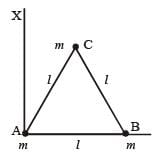Detailed Solution for 31 Year NEET Previous Year Questions: System of Particles & Rotational Motion - 2 - Question 10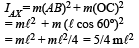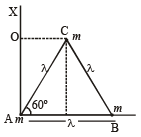31 Year NEET Previous Year Questions: System of Particles & Rotational Motion - 2 - Question 11

The moment of inertia of a uniform circular disc of radius R and mass M about an axis passing from the edge of the disc and normal to the disc is : [2 00 5]

Detailed Solution for 31 Year NEET Previous Year Questions: System of Particles & Rotational Motion - 2 - Question 11

M.I. of a uniform circular disc of radius ‘R’ and mass ‘M’ about an axis passing though C.M. and normal to the disc  is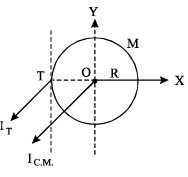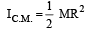From parallel axis theorem,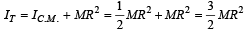31 Year NEET Previous Year Questions: System of Particles & Rotational Motion - 2 - Question 12

​Two bodies have th eir momen ts of in er tia I and 2I respectively about their axis of rotation. If their kinetic energies of rotation are equal, their angular momenta will be in the ratio

Detailed Solution for 31 Year NEET Previous Year Questions: System of Particles & Rotational Motion - 2 - Question 12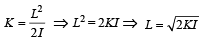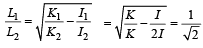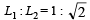31 Year NEET Previous Year Questions: System of Particles & Rotational Motion - 2 - Question 13

A drum of radius R and mass M, rolls down without slipping along an inclined plane of angle θ. The frictional force 

Detailed Solution for 31 Year NEET Previous Year Questions: System of Particles & Rotational Motion - 2 - Question 13

Net work done by frictional force when drum rolls down without slipping is zero.
Wnet = 0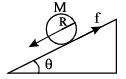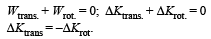i.e., converts translation energy to rotational energy.

31 Year NEET Previous Year Questions: System of Particles & Rotational Motion - 2 - Question 14

The moment of inertia of a uniform circular disc of radius R and mass M about an axis touching the disc at its diameter and normal to the disc is

Detailed Solution for 31 Year NEET Previous Year Questions: System of Particles & Rotational Motion - 2 - Question 14

Moment of Inertia of a disc about an axis perpendicular to its face & passing through its centre is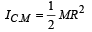Now, given axis is parallel to the axis given above,  so, applying theorem of parallel axis.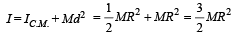31 Year NEET Previous Year Questions: System of Particles & Rotational Motion - 2 - Question 15

A tube of length L is filled completely with an incompressible liquid of mass M and closed at both the ends. The tube is then rotated in a horizontal plane about one of its ends with a uniform angular velocity ω. The force exerted by the liquid at the other end is 

Detailed Solution for 31 Year NEET Previous Year Questions: System of Particles & Rotational Motion - 2 - Question 15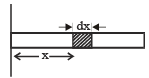Rotation will create centrifugal force due to which pressure will be created at the other end. Now, this centrifugal force will depend on distance of the liquid from the axis as centrifugal force = mω2r.
Let us consider a small part dx of the liquid column which is at a distance of x. Let linear density of liquid be ρ.
Mass of the liquid in dx length = dx.A.ρ (A is cross sectional area of tube) Centrifugal force = dxAρ × ω2x Centrifugal force due to whole of liquid length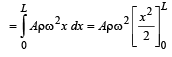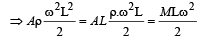31 Year NEET Previous Year Questions: System of Particles & Rotational Motion - 2 - Question 16

A wheel has angular acceleration of 3.0 rad/sec2 and an initial angular speed of 2.00 rad/sec. In a time of 2 sec it has rotated through an angle (in radian) of 

Detailed Solution for 31 Year NEET Previous Year Questions: System of Particles & Rotational Motion - 2 - Question 16

Since,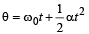Where α is angular aceleration, ωo is the initial angular speed. t = 2s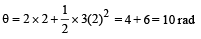31 Year NEET Previous Year Questions: System of Particles & Rotational Motion - 2 - Question 17

A uniform rod AB of length ℓ, and mass m is free to rotate about point A. The rod is released from rest in the horizontal position. Given that the moment of inertia of the rod about A is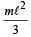, the initial angular acceleration of the rod will be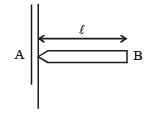Detailed Solution for 31 Year NEET Previous Year Questions: System of Particles & Rotational Motion - 2 - Question 17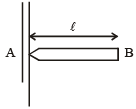Weight of the rod will produce torque,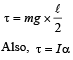where, I is the moment of inertia =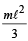and α is the angular acceleration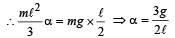31 Year NEET Previous Year Questions: System of Particles & Rotational Motion - 2 - Question 18

A particle of mass m moves in the XY plane with a velocity v along the straight line AB. If the angular momentum of the particle with respect to origin O is LA when it is at A and LB when it is at B, then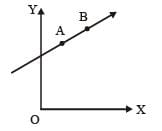Detailed Solution for 31 Year NEET Previous Year Questions: System of Particles & Rotational Motion - 2 - Question 18

Angular momentum = Linear momentum × distance of line of action of linear momentum about the origin.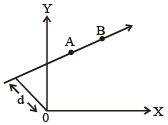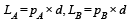As linear momenta are pA and pB  equal, therefore, LA = LB.

31 Year NEET Previous Year Questions: System of Particles & Rotational Motion - 2 - Question 19

The ratio of the radii or gyration of a circular disc to that of a circular ring, each of same mass and radius, around their respective axes is:

Detailed Solution for 31 Year NEET Previous Year Questions: System of Particles & Rotational Motion - 2 - Question 19

The radius of gyration of a circular disc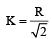The radius of gyration of a circular ring K' = R (Since both have the same mass and radius)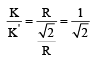31 Year NEET Previous Year Questions: System of Particles & Rotational Motion - 2 - Question 20

A thin rod of length L and mass M is bent at its midpoint into two halves so that the angle between them is 90°. The moment of inertia of the bent rod about an axis passing through the bending point and perpendicular to the plane defined by the two halves of the rod is:   

Detailed Solution for 31 Year NEET Previous Year Questions: System of Particles & Rotational Motion - 2 - Question 20

Mass of each part = M/2 Length of each part = L/2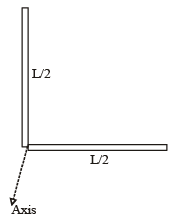Total M.I. = Sum of M.I.s of both parts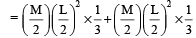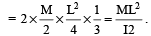31 Year NEET Previous Year Questions: System of Particles & Rotational Motion - 2 - Question 21

A t hin circular ring of mass M an d radius R is rotating in a horizontal plane about an axis vertical to its plane with a constant angular velocity ω. If two objects each of mass m be attached gently to the opposite ends of a diameter of the ring, the ring will then rotate with an angular velocity: 

Detailed Solution for 31 Year NEET Previous Year Questions: System of Particles & Rotational Motion - 2 - Question 21

In absence of exter nal torque, L = Iω  = constant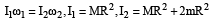(Moment of inertia of a thin circular ring about an axis vertical to its plane = MR2)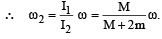31 Year NEET Previous Year Questions: System of Particles & Rotational Motion - 2 - Question 22

If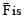the force acting on a particle having position vector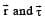be the tor que of this force about the origin, then: 

Detailed Solution for 31 Year NEET Previous Year Questions: System of Particles & Rotational Motion - 2 - Question 22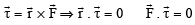Since,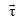is perpendicular to the plane of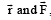, h ence the dot product ofwithis zero.

31 Year NEET Previous Year Questions: System of Particles & Rotational Motion - 2 - Question 23

Four identical th in rods each of mass M and length l, form a square frame. Moment of inertia of this frame about an axis through the centre of the square and perpendicular to its plane is :   

Detailed Solution for 31 Year NEET Previous Year Questions: System of Particles & Rotational Motion - 2 - Question 23

Moment of in ertia of a thin rod of length l about an axis passing through centre and perpendicular to the rod =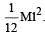Thus moment of inertia of the frame.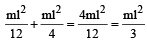Total  M.I.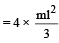31 Year NEET Previous Year Questions: System of Particles & Rotational Motion - 2 - Question 24

Two bodies of mass 1 kg and 3 kg have position vectors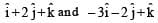ˆ respectively. The centre of mass of this system has a position vector: 

Detailed Solution for 31 Year NEET Previous Year Questions: System of Particles & Rotational Motion - 2 - Question 24

The position vector of the centre of mass of two particle system is given by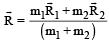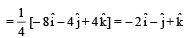31 Year NEET Previous Year Questions: System of Particles & Rotational Motion - 2 - Question 25

A circular disk of moment of inertia It is rotating in a horizontal plane, its symmetry axis, with a constant angular speed ωi . Another disk of moment of inertia Ib is dropped coaxially onto the rotating disk. Initially the second disk has zero angular speed. Eventually both the disks rotate with a constant angular speed ωf . The energy lost by the initially rotating disk to friction is:


Detailed Solution for 31 Year NEET Previous Year Questions: System of Particles & Rotational Motion - 2 - Question 25

By conservation of angular momentum,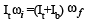where ωf is the final angular velocity ofdisks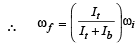Loss in K.E., ΔK = Initial K.E. – Final K.E.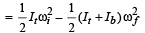⇒ ΔK =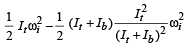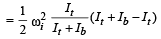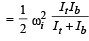31 Year NEET Previous Year Questions: System of Particles & Rotational Motion - 2 - Question 26

Two particles which are initially at rest, move towards each other under the action of their internal attraction. If their speeds are v and 2v at any instant, then the speed of centre of mass of the system will be: 

Detailed Solution for 31 Year NEET Previous Year Questions: System of Particles & Rotational Motion - 2 - Question 26

If n o extern al for ce actson a system of particles, the centre of mass remains at rest.
So, speed of centre of mass is zero.

31 Year NEET Previous Year Questions: System of Particles & Rotational Motion - 2 - Question 27

A gramophone recor d is revolving with an angular velocity ω. A coin is placed at a distance r from the centre of the record. The static coefficient of friction is ω. The coin will revolve with the record if: 

Detailed Solution for 31 Year NEET Previous Year Questions: System of Particles & Rotational Motion - 2 - Question 27

For the coin to revolve with the record, centrifugal force ≤ frictional force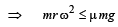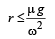31 Year NEET Previous Year Questions: System of Particles & Rotational Motion - 2 - Question 28

The instantaneous angular position of a point on a rotating wheel is given by the equation θ(t) = 2t3 – 6t2. The torque on the wheel becomes zero at

Detailed Solution for 31 Year NEET Previous Year Questions: System of Particles & Rotational Motion - 2 - Question 28

When angular acceleration (α) is zero then torque on the wheel becomes zero. θ(t) = 2t3 – 6t2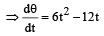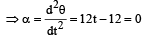∴  t = 1 sec.

31 Year NEET Previous Year Questions: System of Particles & Rotational Motion - 2 - Question 29

The moment of inertia of a thin uniform rod of mass M and length L about an axis passing through its midpoint and perpendicular to its length is I0. Its moment of inertia about an axis passing through one of its ends and perpendicular to its length is 

Detailed Solution for 31 Year NEET Previous Year Questions: System of Particles & Rotational Motion - 2 - Question 29

By theorem of parallel axes,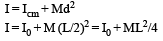31 Year NEET Previous Year Questions: System of Particles & Rotational Motion - 2 - Question 30

A small mass attached to a string rotates on frictionless table top as shown. If the tension in the string is increased by pulling the string causing the radius of the circular motion to decrease by a factor of 2, the kinetic energy of the mass will [2011M]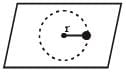Detailed Solution for 31 Year NEET Previous Year Questions: System of Particles & Rotational Motion - 2 - Question 30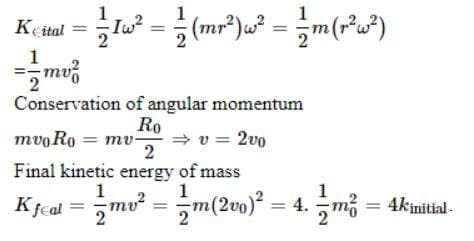## Physics Class 11

127 videos|464 docs|210 tests
 Use Code STAYHOME200 and get INR 200 additional OFF Use Coupon Code
Information about 31 Year NEET Previous Year Questions: System of Particles & Rotational Motion - 2 Page
In this test you can find the Exam questions for 31 Year NEET Previous Year Questions: System of Particles & Rotational Motion - 2 solved & explained in the simplest way possible. Besides giving Questions and answers for 31 Year NEET Previous Year Questions: System of Particles & Rotational Motion - 2, EduRev gives you an ample number of Online tests for practice

## Physics Class 11

127 videos|464 docs|210 tests

### How to Prepare for NEET

Read our guide to prepare for NEET which is created by Toppers & the best Teachers